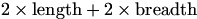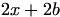### Rocky

Posted 01 December 2005 - 06:05 PM

Q- A rectangular field is 'x' metres long. It is enclosed by 200 metres of fencing.
(a) Find an expression in terms of 'x' for the breadth of the field
(b) Hence find the greatest area which can be enclosed with the 200 metres of fencing.

*Can someone tell me what I need to do, to solve this Q please

### Steve

Posted 01 December 2005 - 07:04 PM

I'm not going to tell you the answer, but for part (a) you are told that the perimeter is 200 m and that the length is x m.

Let b be the breadth of the field, and then find a formula for the perimiter.

If you can't see how to go on from there, just post again.### Rocky

Posted 02 December 2005 - 02:16 PM

I don't know how to do it, so can you help me.

### dfx

Posted 02 December 2005 - 04:19 PM

Part (a) is probably S1/S2 level. Especially with that hint that George gave.

### Dave

Posted 02 December 2005 - 05:05 PM

part a) the whole perimeter is 200m, so the total is 200. two sides we know is "x". Our job is to find the other side. So if we have 2 sides at length x what will the other two side be to give a total of 200

part b

Finding largest area is differentiation

### Steve

Posted 02 December 2005 - 09:10 PM

It's actually differentiation.### Steve

Posted 02 December 2005 - 09:16 PM

OK, so we have a rectangle, whose perimeter is 200 m, and the length of one side is x m.

The perimeter is given byand you know that the length is x m and you want to find the breadth, call it b m.

So the perimeter ism and you also know it's 200 m. So:So the breadth in terms of x ismetres. And that's part (a) done.

### Dave

Posted 02 December 2005 - 10:17 PM

QUOTE(Steve @ Dec 2 2005, 09:10 PM)
It's actually differentiation.och i thought it was differentiation but i somehow convinced myself it was integration

either way i have editted it on my post

### Rocky

Posted 03 December 2005 - 12:50 PM

Q- A curve is such that its derivative is defined as dy/dx= x - (1/x-squared)
If the curve passes through the point (2, -5/2), find the eqn of the curve.

*Do I sub in the x-coord into the dy/dx eqn. Then go onto solve, or is this wrong?

### John

Posted 03 December 2005 - 12:58 PM

You use integration on the derivitive, then subsitute the x and y values into the integral to find C, then rewrite the equation with value for C which you just found

### Rocky

Posted 03 December 2005 - 06:22 PM

I intergrated the Eqn and I got:
1/2(x-squared) + x(power -1) + C

*Is this right dude? Btw how can I sub Y-values into it? After I sub X-values into it, what do I do?

### Katrina

Posted 03 December 2005 - 06:30 PM

because the equation you get when you integrate is equal to y, since when you integrate you need to remember to do both sides so integrating dy/dx gives you y

### Rocky

Posted 03 December 2005 - 06:36 PM

I see.

### Rocky

Posted 03 December 2005 - 06:41 PM

QUOTE(Steve @ Dec 2 2005, 10:16 PM)
OK, so we have a rectangle, whose perimeter is 200 m, and the length of one side is x m.

The perimeter is given byand you know that the length is x m and you want to find the breadth, call it b m.

So the perimeter ism and you also know it's 200 m. So:So the breadth in terms of x ismetres. And that's part (a) done.I then differentiated:
100x - (x-squared)

And it gave me x=100, is this correct?

### John

Posted 03 December 2005 - 07:44 PM

Ignore me, i should learn to readEdited by John, 03 December 2005 - 09:01 PM.

### Rocky

Posted 03 December 2005 - 08:28 PM

x - (1/x squared) dx
x- (x minus squared) dx
(x squared/2) - (x-1/-1) + C
1/2(x squared) + x(power -1)